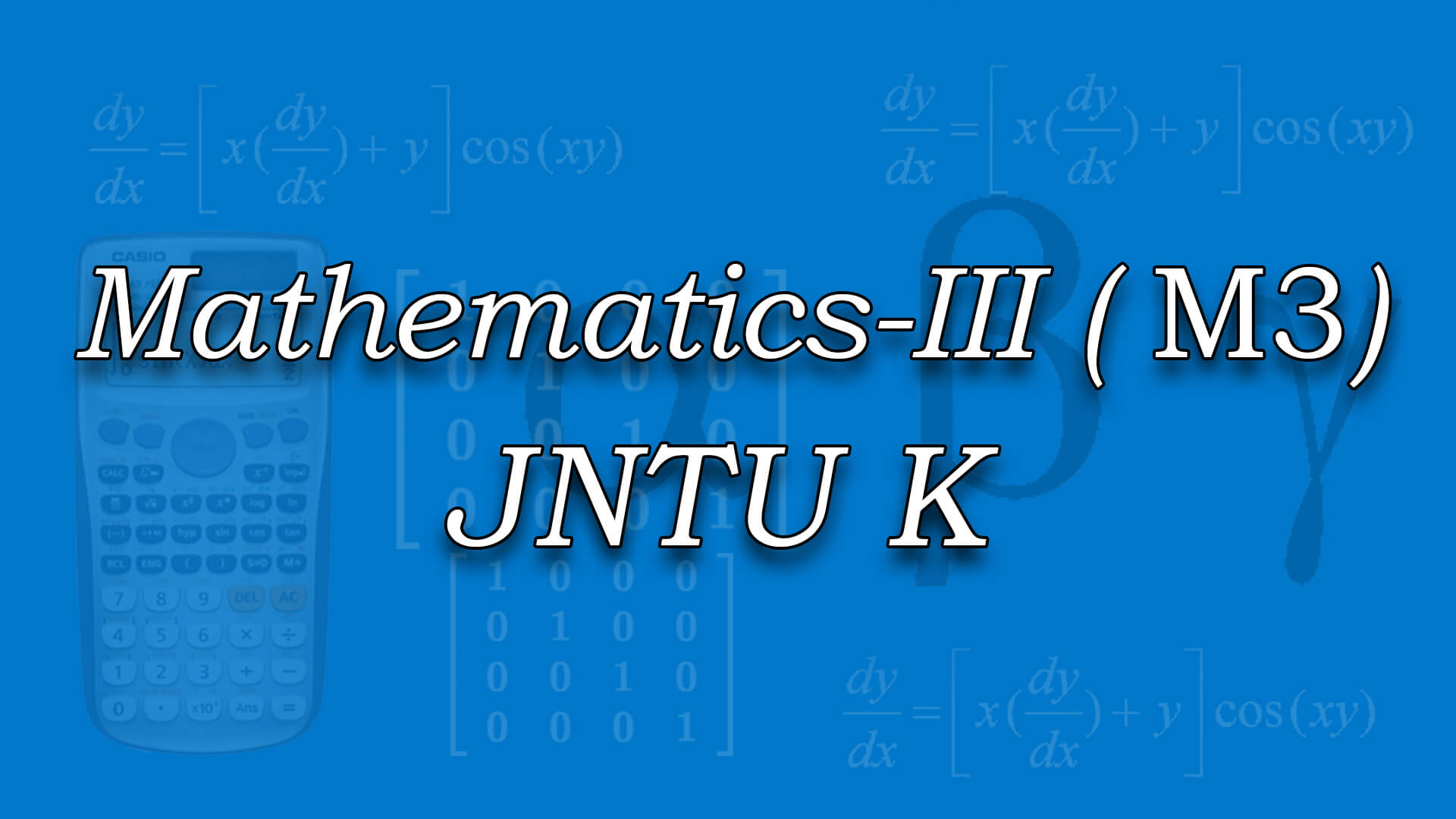## Mathematics-III for JNTUK by Dr C N B Rao ., M.Sc ,Ph.D (IIT Kharagpur ) The course is designed to equip the students with the necessary mathematical skills and techniques that are essential for an engineering course. The skills derived from the course will help the student from a necessary base to develop analytic and design concepts. Code : M3JNTUK Recommended For : B.Tech 1st Year 2nd Sem JNTUK

#### After Learning this subject,you should be able to

• Determine rank, Eigenvalues and Eigen vectors of a given matrix and solve simultaneous linear equations.
• Solve simultaneous linear equations numerically using various matrix methods..
• Determine double integral over a region and triple integral over a volume.
• Calculate gradient of a scalar function, divergence and curl of a vector function. Determine line, surface and volume integrals. Apply Green, Stokes and Gauss divergence theorems to calculate line, surface and volume integrals.

## Curriculum 10:03:49   units   |   6

• 1:44:36

UNIT 1
Linear Systems of Equations: Introduction, Rank, Echelon form, Normal form- Part 1, Normal form- Part 2, Gauss-Jordan method of finding inverse of a matrix, Solution of linear systems, Solution of linear systems Part 1, Gauss Elimination, Gauss-Jordan, Jacobi and Gauss Seidel Method, Jacobi and Gauss Seidel Method Part 1, Applications : Finding the current in electrical circuits

• 2:08:14

UNIT 2
Eigen values-Eigen Vectors and Quadratic forms: Eigen Values and Eigen vectors, Properties, Problems on Eigen Values and Eigen vectors, Problems on Eigen Values and Eigen vectors Part 1, Cayley-Hamilton theorem, Problems on Cayley-Hamilton theorem, Problem Inverse and Powers of matrix by using Cayley-Hamilton theorem, Diagonalization, Problem on Diagonalization, Quadratic forms, Reduction of a Quadratic form to Canonical form, Problems on Reduction of a Quadratic form to Canonical form Part 1, Problems on Reduction of a Quadratic form to Canonical form Part 2, Problems on Reduction of a Quadratic form to Canonical form Part 3, Problems on Reduction of a Quadratic form to Canonical form Part 4, Rank , Positive,Negative forms Quadratic form, Semi-definite and indefinite forms of Quadratic form, Index and Signature of a quadratic form, Applications: Free Vibration of a two-mass system

• 1:40:55

UNIT 3
Multiple Integrals: Introduction, Double Integrals, Triple Integrals, Triple Integrals Part 1, Change of variables, Change of order of integration, Applications to find Areas, Applications to find Areas Part 1, Moment of Inertia and volumes, Moment of Inertia and volumes Part 1

• 1:04:12

UNIT 4
Special Functions: Gamma Function, Gamma Function Part 1, Beta Function, Properties, Relation between Beta and Gamma functions, Relation between Beta and Gamma functions - Part 1, Relation between Beta and Gamma functions - Part 2, Applications to evaluation of improper integrals, The error function & Complimentory error function

• 1:58:44

UNIT 5
Vector Differentiation: Introduction to Vector Differentiation, Gradient, Directional derivative, Problems on Gradient and Directional Derivative - Part 1, Problems on Gradient and Directional Derivative - Part 2, Divergence, Curl, Problems on Gradient,Divergence and Curl, Problems on Gradient,Divergence and Curl Part 1, Incompressible flow, Solenoidal and irrotational vector fields, Second order Operators, Del applied twice to point function, Del applied twice to point function Problems, Del applied twice to point function Problems Part 1, Del applied to products of point functions, Problems on Del applied to products of point functions, Problems on Del applied to products of point functions Part 1, Vector identities

• 1:27:08

UNIT 6
Vector Integration: Line Integral, Problems On Work Done, Problems On Work Done - Part 1, Potential Function, Area,surface and volume of integrals, Flux, Vector integral theorems, Greens Theorem, Green's Theorem Problems, Stokes Theorem, Stokes Theorem Part 1, Stokes Theorem Part 2, Gauss Divergence theorem, Gauss Divergence theorem Part 1